Скачать презентацию Introduction to Lossless Compression Trac D Tran ECE

• Количество слайдов: 38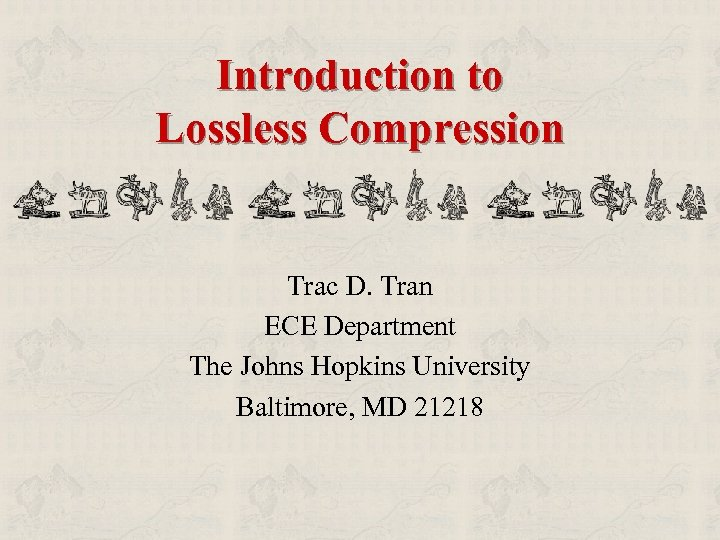Introduction to Lossless Compression Trac D. Tran ECE Department The Johns Hopkins University Baltimore, MD 21218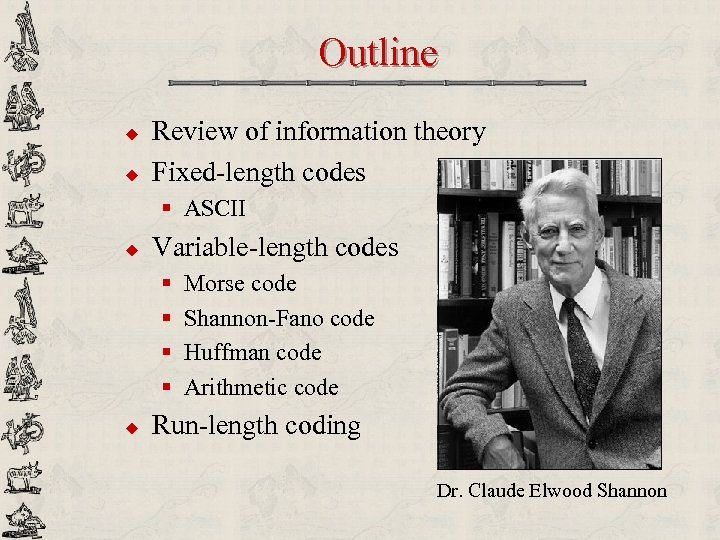Outline u u Review of information theory Fixed-length codes § ASCII u Variable-length codes § § u Morse code Shannon-Fano code Huffman code Arithmetic code Run-length coding Dr. Claude Elwood Shannon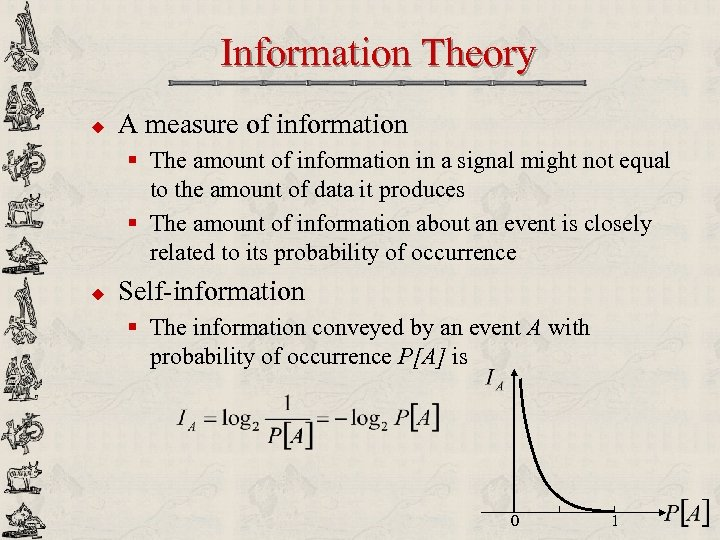Information Theory u A measure of information § The amount of information in a signal might not equal to the amount of data it produces § The amount of information about an event is closely related to its probability of occurrence u Self-information § The information conveyed by an event A with probability of occurrence P[A] is 0 1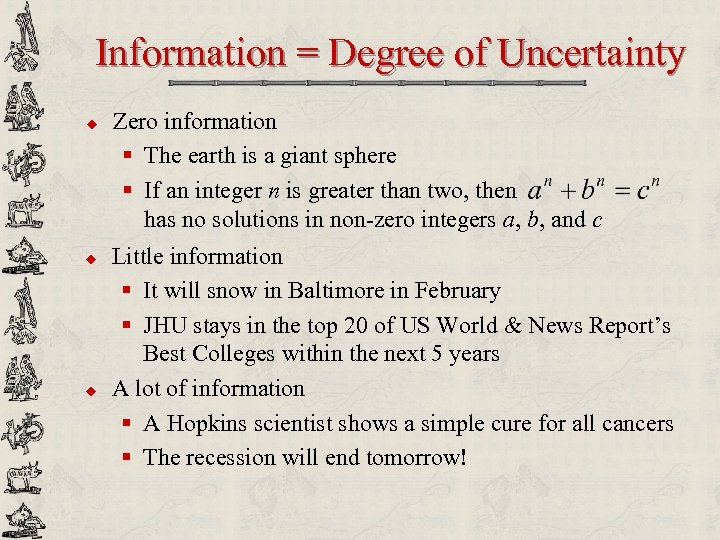Information = Degree of Uncertainty u u u Zero information § The earth is a giant sphere § If an integer n is greater than two, then has no solutions in non-zero integers a, b, and c Little information § It will snow in Baltimore in February § JHU stays in the top 20 of US World & News Report’s Best Colleges within the next 5 years A lot of information § A Hopkins scientist shows a simple cure for all cancers § The recession will end tomorrow!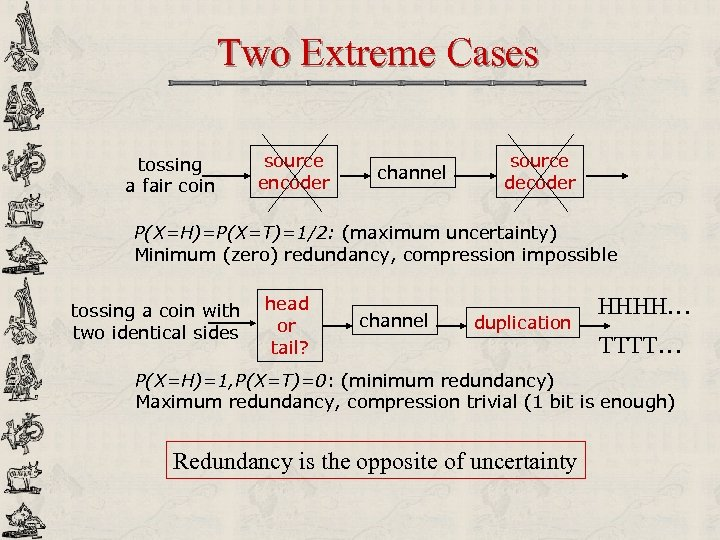Two Extreme Cases tossing a fair coin source encoder channel source decoder P(X=H)=P(X=T)=1/2: (maximum uncertainty) Minimum (zero) redundancy, compression impossible tossing a coin with two identical sides head or tail? channel duplication HHHH… TTTT… P(X=H)=1, P(X=T)=0: (minimum redundancy) Maximum redundancy, compression trivial (1 bit is enough) Redundancy is the opposite of uncertainty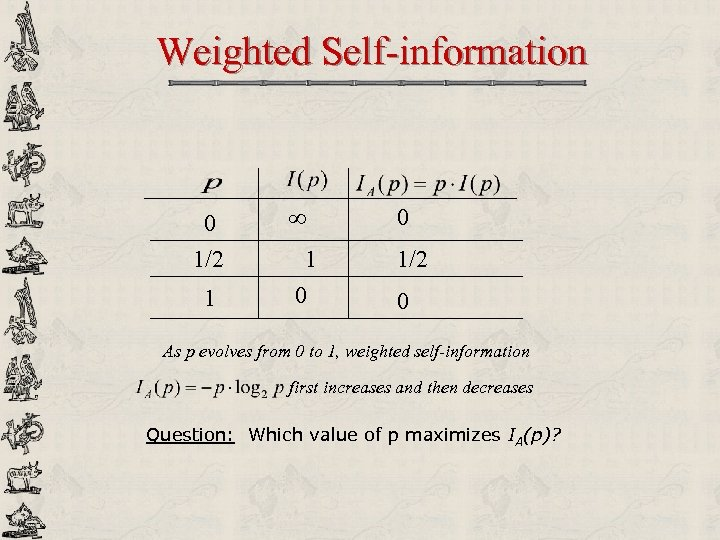Weighted Self-information 0 1/2 1 0 1/2 0 As p evolves from 0 to 1, weighted self-information first increases and then decreases Question: Which value of p maximizes IA(p)?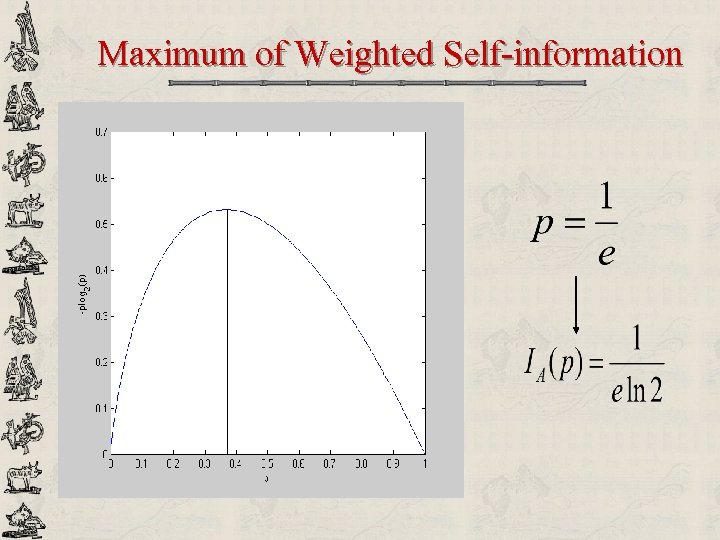Maximum of Weighted Self-information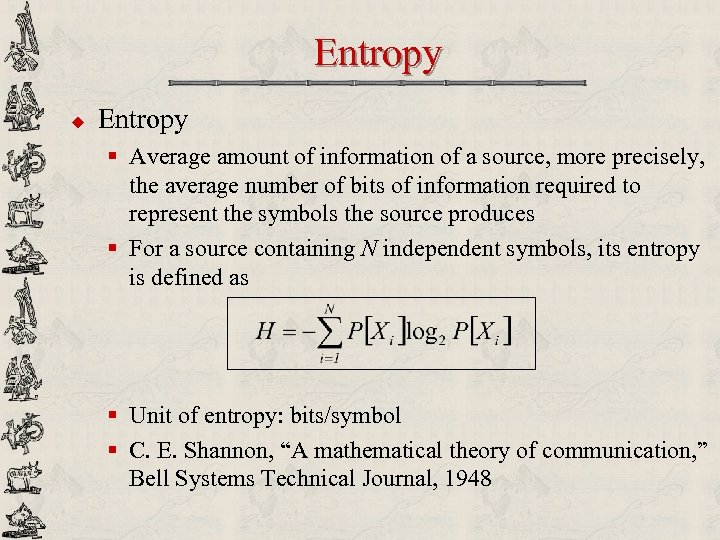Entropy u Entropy § Average amount of information of a source, more precisely, the average number of bits of information required to represent the symbols the source produces § For a source containing N independent symbols, its entropy is defined as § Unit of entropy: bits/symbol § C. E. Shannon, “A mathematical theory of communication, ” Bell Systems Technical Journal, 1948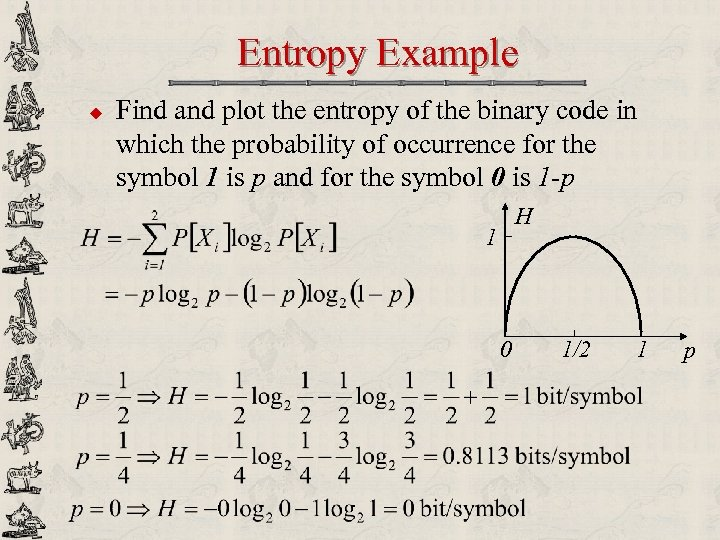Entropy Example u Find and plot the entropy of the binary code in which the probability of occurrence for the symbol 1 is p and for the symbol 0 is 1 -p H 1 0 1/2 1 p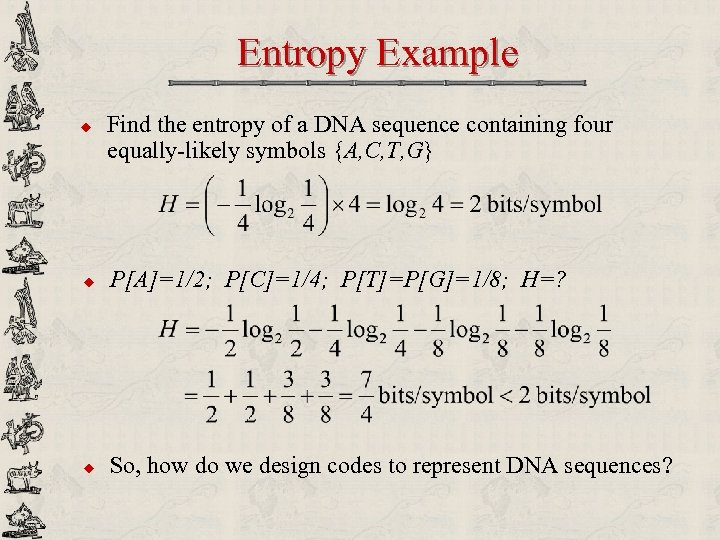Entropy Example u Find the entropy of a DNA sequence containing four equally-likely symbols {A, C, T, G} u P[A]=1/2; P[C]=1/4; P[T]=P[G]=1/8; H=? u So, how do we design codes to represent DNA sequences?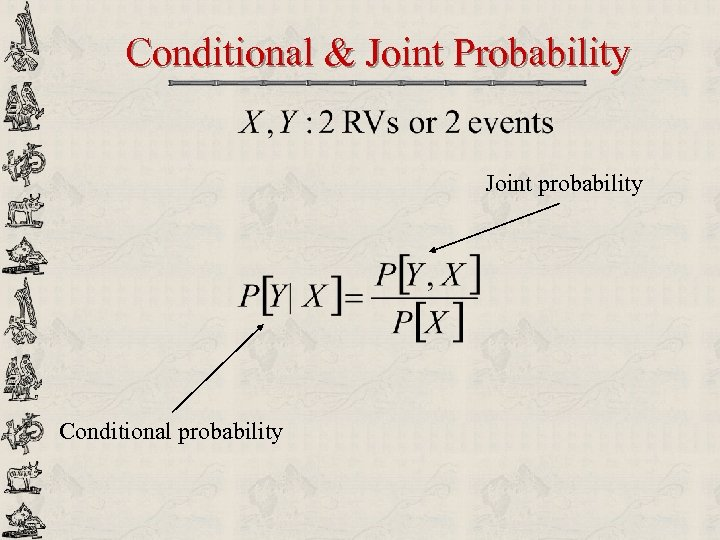Conditional & Joint Probability Joint probability Conditional probability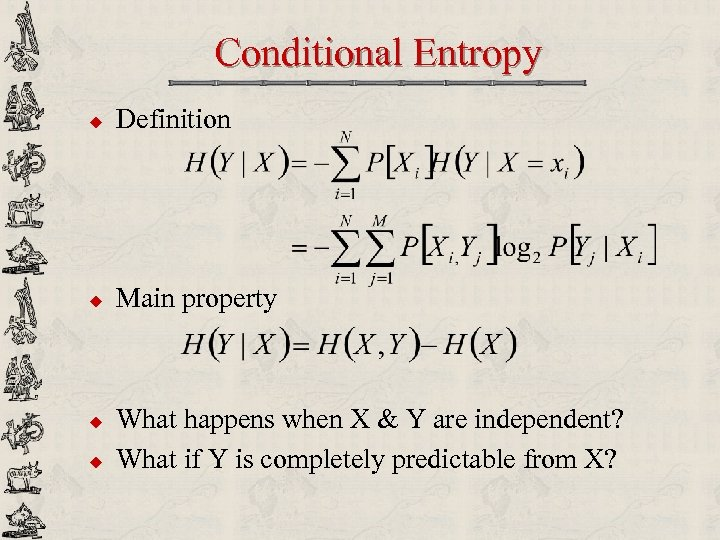Conditional Entropy u Definition u Main property u u What happens when X & Y are independent? What if Y is completely predictable from X?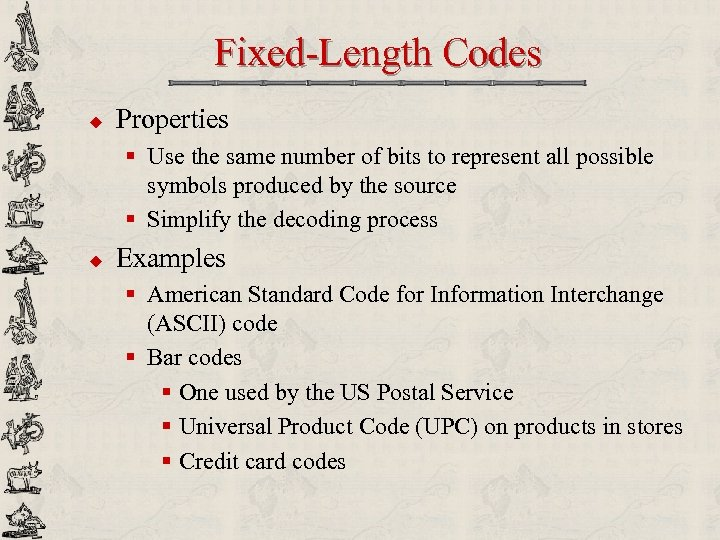Fixed-Length Codes u Properties § Use the same number of bits to represent all possible symbols produced by the source § Simplify the decoding process u Examples § American Standard Code for Information Interchange (ASCII) code § Bar codes § One used by the US Postal Service § Universal Product Code (UPC) on products in stores § Credit card codes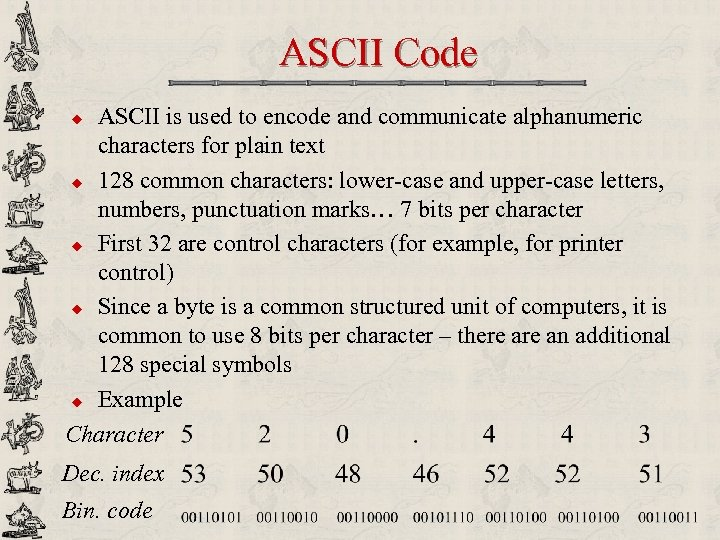ASCII Code ASCII is used to encode and communicate alphanumeric characters for plain text u 128 common characters: lower-case and upper-case letters, numbers, punctuation marks… 7 bits per character u First 32 are control characters (for example, for printer control) u Since a byte is a common structured unit of computers, it is common to use 8 bits per character – there an additional 128 special symbols u Example Character u Dec. index Bin. code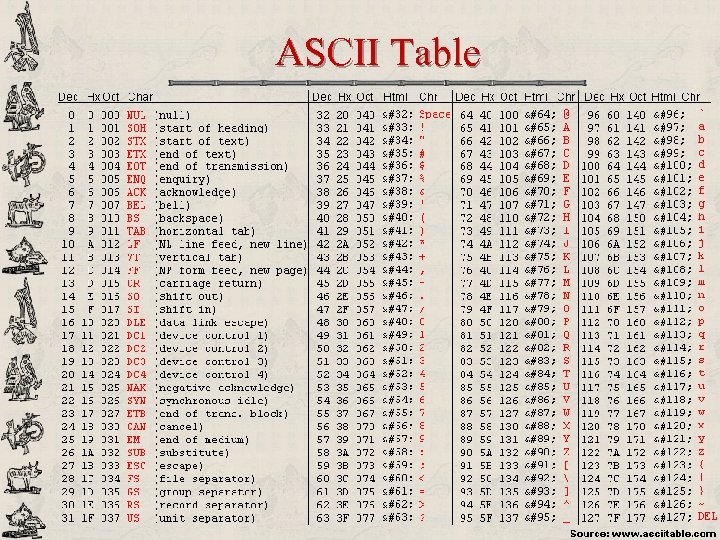ASCII Table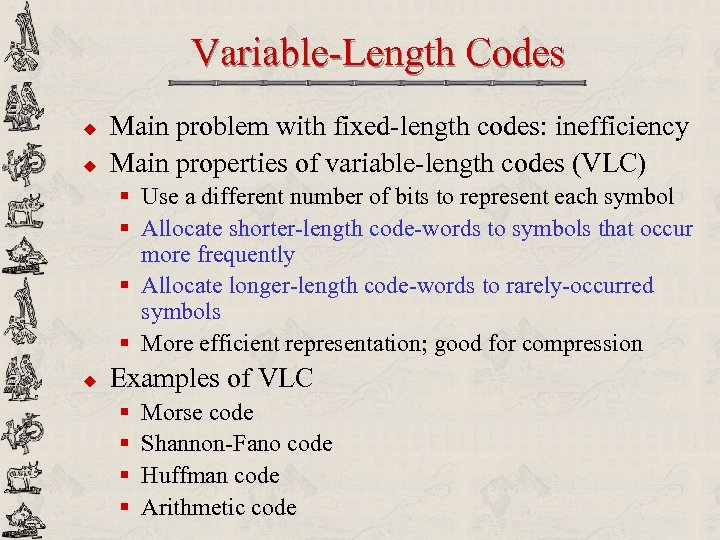Variable-Length Codes u u Main problem with fixed-length codes: inefficiency Main properties of variable-length codes (VLC) § Use a different number of bits to represent each symbol § Allocate shorter-length code-words to symbols that occur more frequently § Allocate longer-length code-words to rarely-occurred symbols § More efficient representation; good for compression u Examples of VLC § § Morse code Shannon-Fano code Huffman code Arithmetic code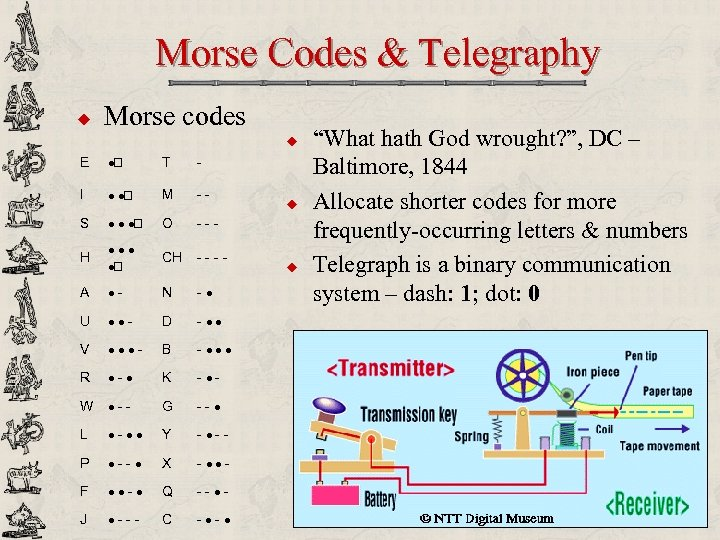Morse Codes & Telegraphy u Morse codes u E · T - I · · M -- S · · · O --- H ··· · CH ---- A ·- N -· U ··- D -·· V ···- B -··· R ·-· K -·- W ·-- G --· L ·-·· Y -·-- P ·--· X -··- F ··-· Q --·- J ·--- C -·-· u u “What hath God wrought? ”, DC – Baltimore, 1844 Allocate shorter codes for more frequently-occurring letters & numbers Telegraph is a binary communication system – dash: 1; dot: 0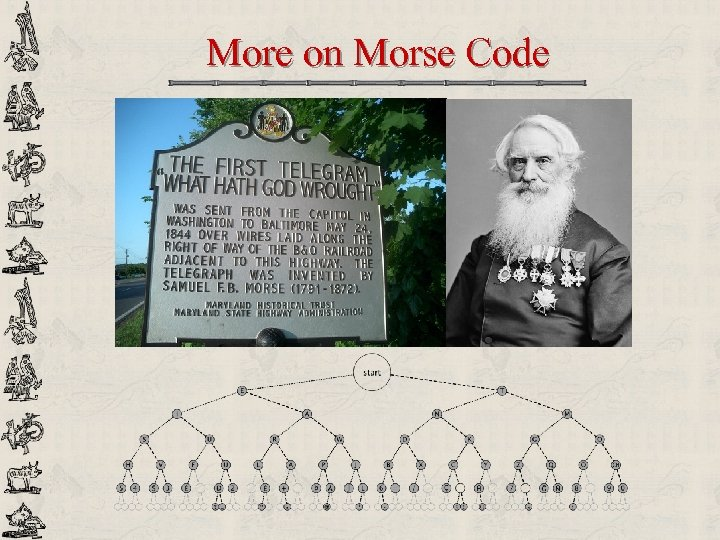More on Morse Code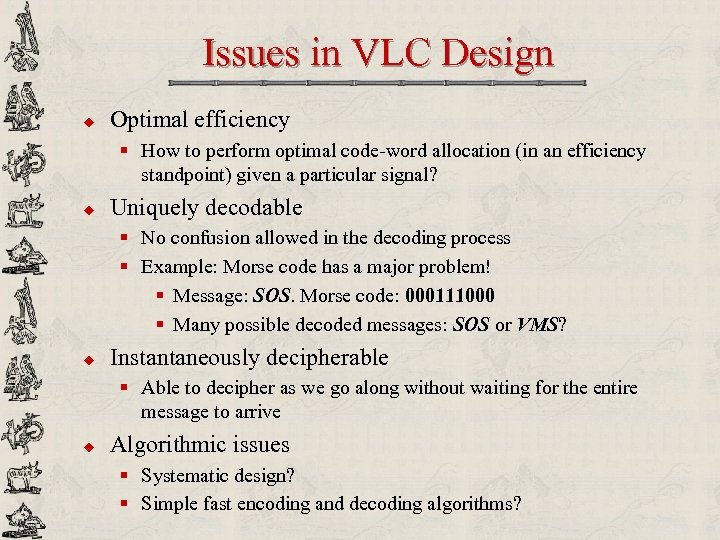Issues in VLC Design u Optimal efficiency § How to perform optimal code-word allocation (in an efficiency standpoint) given a particular signal? u Uniquely decodable § No confusion allowed in the decoding process § Example: Morse code has a major problem! § Message: SOS. Morse code: 000111000 § Many possible decoded messages: SOS or VMS? u Instantaneously decipherable § Able to decipher as we go along without waiting for the entire message to arrive u Algorithmic issues § Systematic design? § Simple fast encoding and decoding algorithms?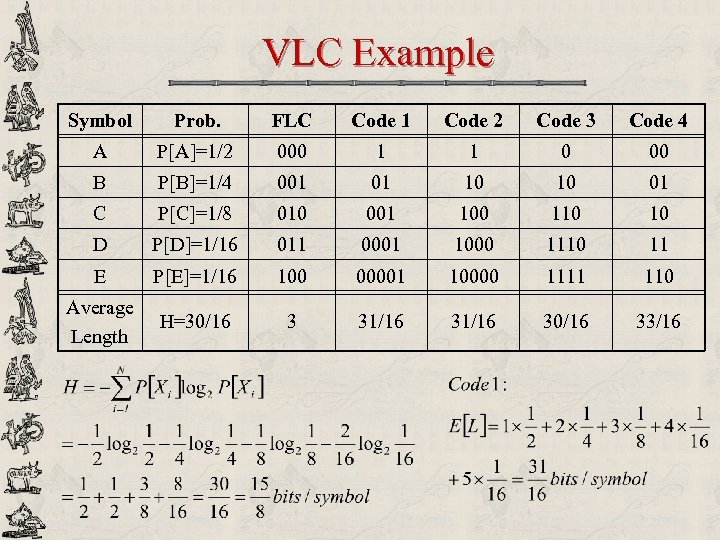VLC Example Symbol Prob. FLC Code 1 Code 2 Code 3 Code 4 A P[A]=1/2 000 1 1 0 00 B P[B]=1/4 001 01 10 10 01 C P[C]=1/8 010 001 100 110 10 D P[D]=1/16 011 0001 1000 1110 11 E P[E]=1/16 100 00001 10000 1111 110 Average Length H=30/16 3 31/16 30/16 33/16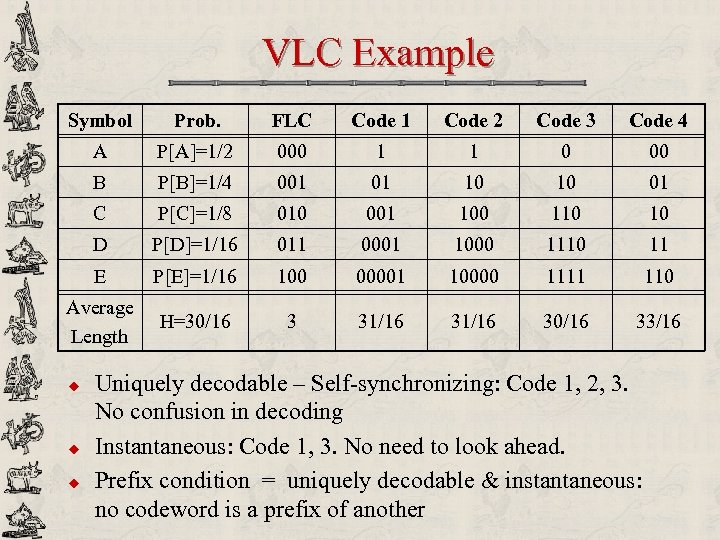VLC Example Symbol Prob. FLC Code 1 Code 2 Code 3 Code 4 A P[A]=1/2 000 1 1 0 00 B P[B]=1/4 001 01 10 10 01 C P[C]=1/8 010 001 100 110 10 D P[D]=1/16 011 0001 1000 1110 11 E P[E]=1/16 100 00001 10000 1111 110 Average Length H=30/16 3 31/16 30/16 33/16 u u u Uniquely decodable – Self-synchronizing: Code 1, 2, 3. No confusion in decoding Instantaneous: Code 1, 3. No need to look ahead. Prefix condition = uniquely decodable & instantaneous: no codeword is a prefix of another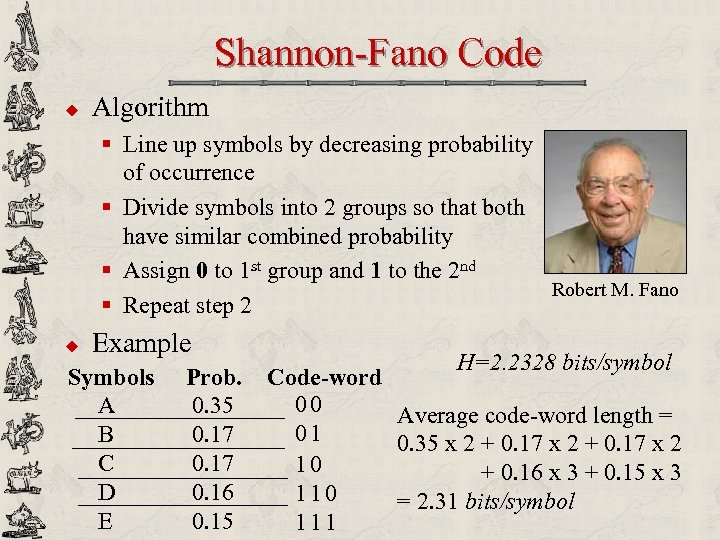Shannon-Fano Code u Algorithm § Line up symbols by decreasing probability of occurrence § Divide symbols into 2 groups so that both have similar combined probability § Assign 0 to 1 st group and 1 to the 2 nd Robert M. Fano § Repeat step 2 u Example Symbols A B C D E Prob. 0. 35 0. 17 0. 16 0. 15 H=2. 2328 bits/symbol Code-word 00 Average code-word length = 01 0. 35 x 2 + 0. 17 x 2 10 + 0. 16 x 3 + 0. 15 x 3 110 = 2. 31 bits/symbol 111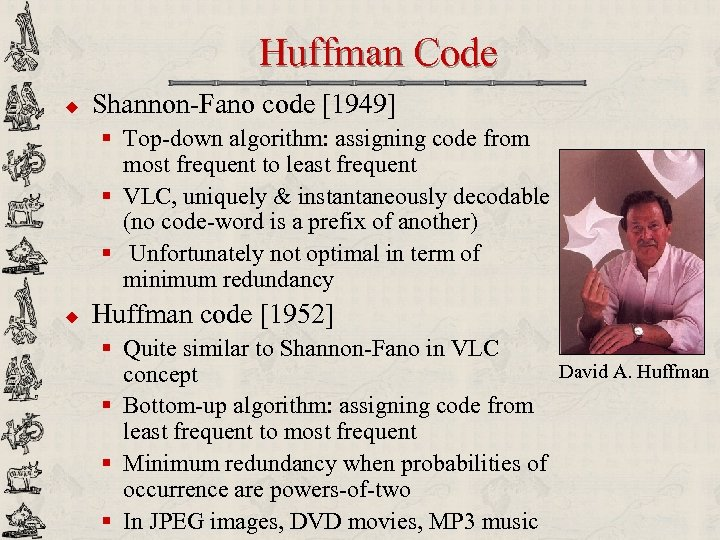Huffman Code u Shannon-Fano code  § Top-down algorithm: assigning code from most frequent to least frequent § VLC, uniquely & instantaneously decodable (no code-word is a prefix of another) § Unfortunately not optimal in term of minimum redundancy u Huffman code  § Quite similar to Shannon-Fano in VLC David A. Huffman concept § Bottom-up algorithm: assigning code from least frequent to most frequent § Minimum redundancy when probabilities of occurrence are powers-of-two § In JPEG images, DVD movies, MP 3 music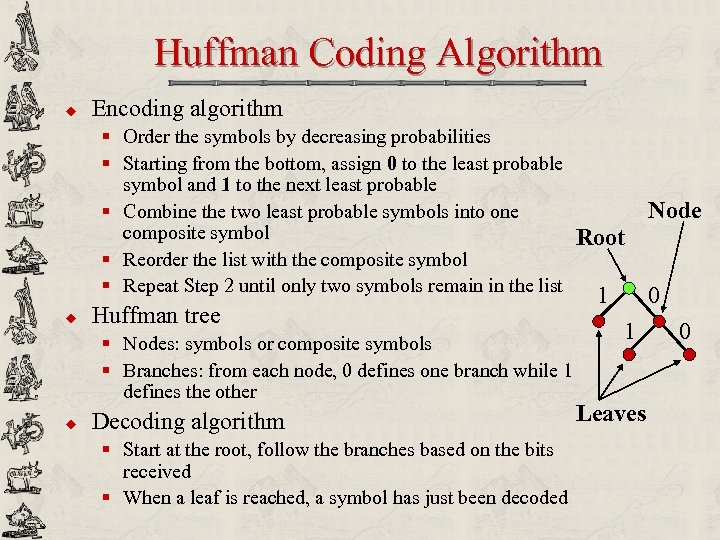Huffman Coding Algorithm u Encoding algorithm § Order the symbols by decreasing probabilities § Starting from the bottom, assign 0 to the least probable symbol and 1 to the next least probable § Combine the two least probable symbols into one composite symbol Root § Reorder the list with the composite symbol § Repeat Step 2 until only two symbols remain in the list u Huffman tree § Nodes: symbols or composite symbols § Branches: from each node, 0 defines one branch while 1 defines the other u Decoding algorithm § Start at the root, follow the branches based on the bits received § When a leaf is reached, a symbol has just been decoded 1 1 Leaves Node 0 0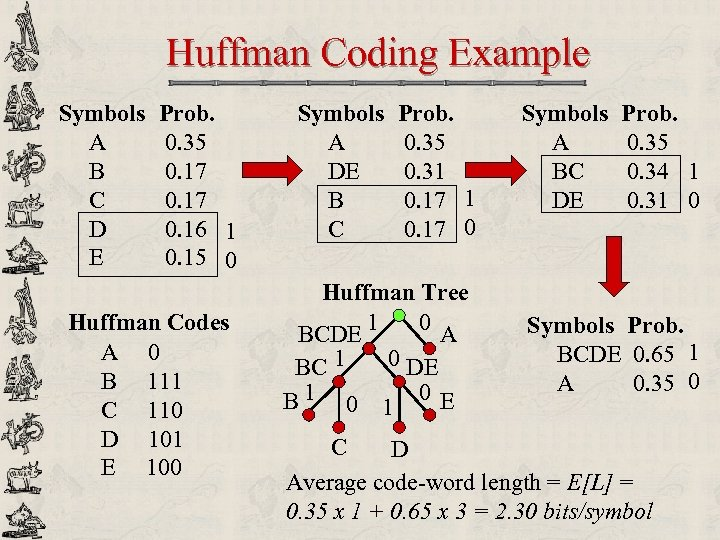Huffman Coding Example Symbols A B C D E Prob. 0. 35 0. 17 0. 16 1 0. 15 0 Huffman Codes A 0 B 111 C 110 D 101 E 100 Symbols A DE B C Prob. 0. 35 0. 31 0. 17 0 Huffman Tree 1 0 BCDE A 0 DE BC 1 B 1 0 E Symbols A BC DE Prob. 0. 35 0. 34 1 0. 31 0 Symbols Prob. BCDE 0. 65 1 A 0. 35 0 C D Average code-word length = E[L] = 0. 35 x 1 + 0. 65 x 3 = 2. 30 bits/symbol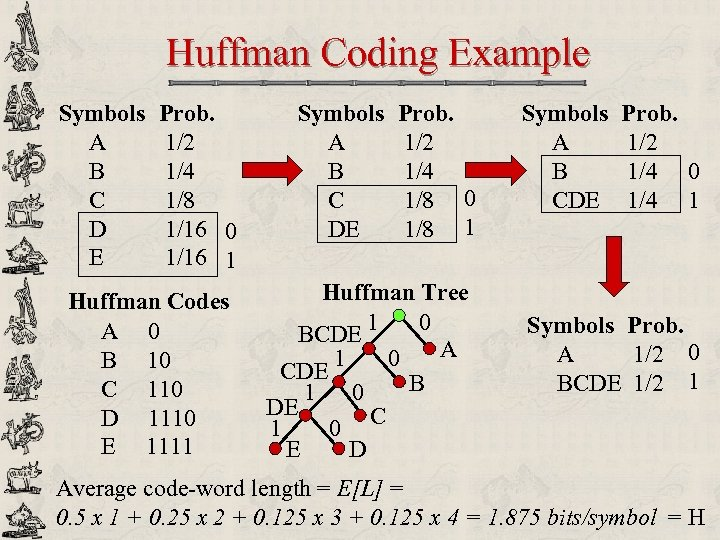Huffman Coding Example Symbols A B C D E Prob. 1/2 1/4 1/8 1/16 0 1/16 1 Huffman Codes A 0 B 10 C 110 D 1110 E 1111 Symbols A B C DE Prob. 1/2 1/4 1/8 0 1/8 1 Huffman Tree 1 0 BCDE A 1 0 CDE B 1 0 DE C 1 0 D E Symbols A B CDE Prob. 1/2 1/4 0 1/4 1 Symbols Prob. A 1/2 0 BCDE 1/2 1 Average code-word length = E[L] = 0. 5 x 1 + 0. 25 x 2 + 0. 125 x 3 + 0. 125 x 4 = 1. 875 bits/symbol = H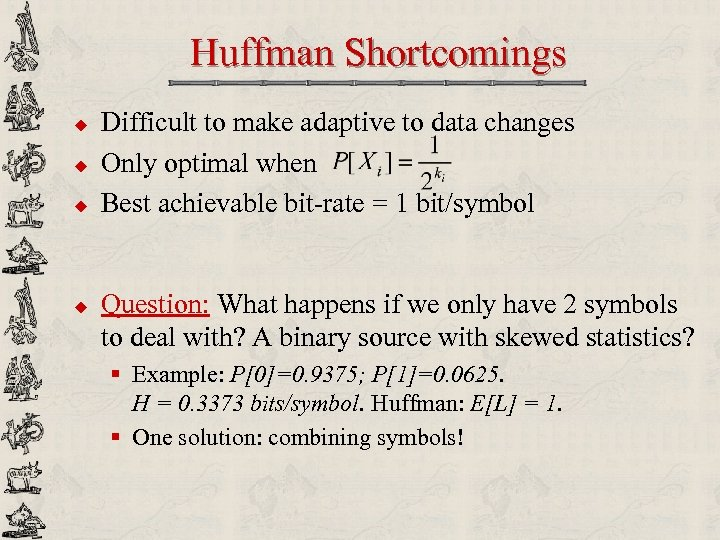Huffman Shortcomings u u Difficult to make adaptive to data changes Only optimal when Best achievable bit-rate = 1 bit/symbol Question: What happens if we only have 2 symbols to deal with? A binary source with skewed statistics? § Example: P=0. 9375; P=0. 0625. H = 0. 3373 bits/symbol. Huffman: E[L] = 1. § One solution: combining symbols!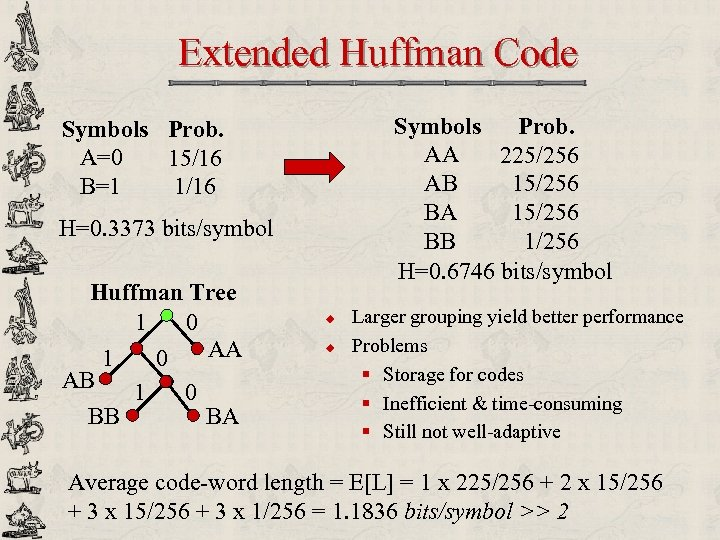Extended Huffman Code Symbols Prob. AA 225/256 AB 15/256 BA 15/256 BB 1/256 H=0. 6746 bits/symbol Symbols Prob. A=0 15/16 B=1 1/16 H=0. 3373 bits/symbol Huffman Tree 1 0 AA 1 0 AB 1 0 BB BA u u Larger grouping yield better performance Problems § Storage for codes § Inefficient & time-consuming § Still not well-adaptive Average code-word length = E[L] = 1 x 225/256 + 2 x 15/256 + 3 x 1/256 = 1. 1836 bits/symbol >> 2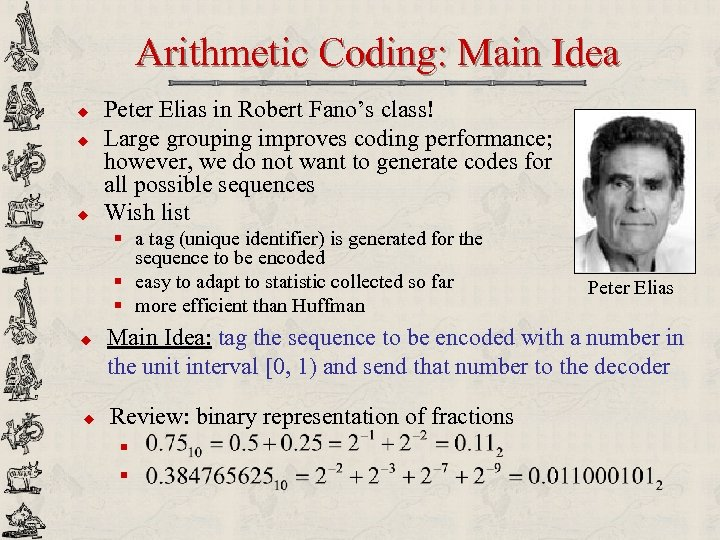Arithmetic Coding: Main Idea u u u Peter Elias in Robert Fano’s class! Large grouping improves coding performance; however, we do not want to generate codes for all possible sequences Wish list § a tag (unique identifier) is generated for the sequence to be encoded § easy to adapt to statistic collected so far § more efficient than Huffman u u Peter Elias Main Idea: tag the sequence to be encoded with a number in the unit interval [0, 1) and send that number to the decoder Review: binary representation of fractions § §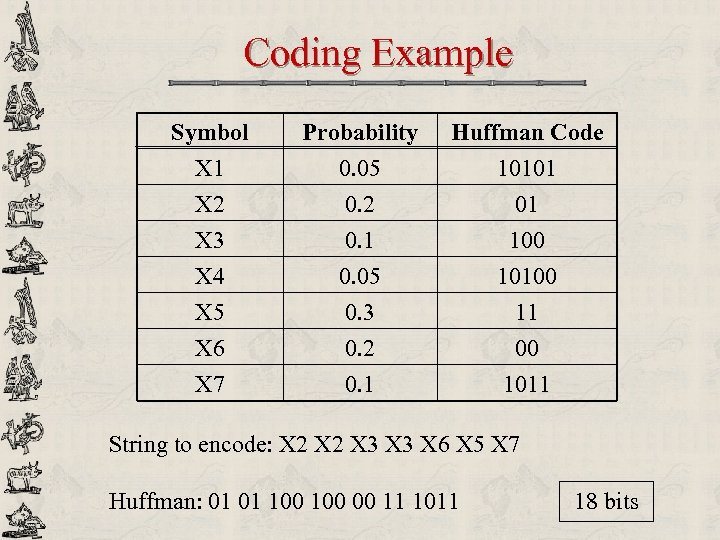Coding Example Symbol X 1 X 2 X 3 Probability 0. 05 0. 2 0. 1 Huffman Code 10101 01 100 X 4 X 5 X 6 X 7 0. 05 0. 3 0. 2 0. 1 10100 11 00 1011 String to encode: X 2 X 3 X 6 X 5 X 7 Huffman: 01 01 100 00 11 1011 18 bits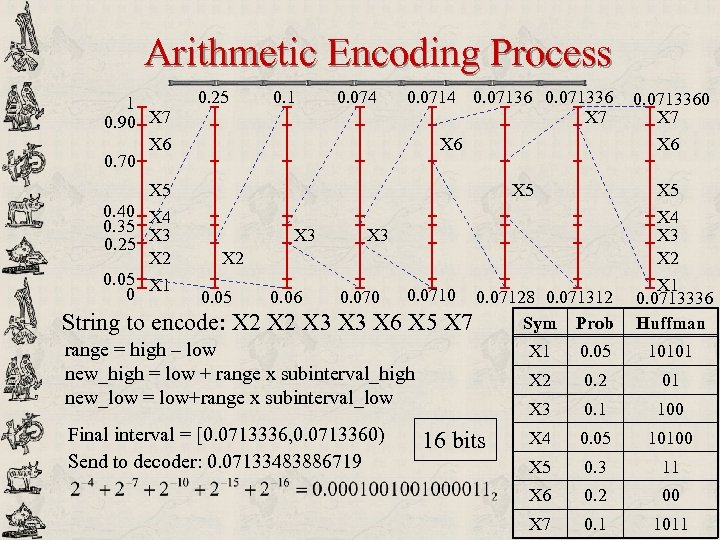Arithmetic Encoding Process 1 0. 90 X 7 X 6 0. 70 0. 25 0. 1 0. 074 0. 07136 0. 071336 X 7 X 6 X 5 0. 40 X 4 0. 35 X 3 0. 25 X 2 0. 05 X 1 0 X 6 X 5 X 3 X 5 X 4 X 3 X 2 0. 05 0. 06 0. 070 0. 0710 String to encode: X 2 X 3 X 6 X 5 0. 07128 0. 071312 Sym Prob X 7 range = high – low new_high = low + range x subinterval_high new_low = low+range x subinterval_low Final interval = [0. 0713336, 0. 0713360) Send to decoder: 0. 07133483886719 0. 0713360 X 7 X 1 0. 0713336 Huffman X 1 10101 X 2 01 X 3 16 bits 0. 05 0. 1 100 X 4 0. 05 10100 X 5 0. 3 11 X 6 0. 2 00 X 7 0. 1 1011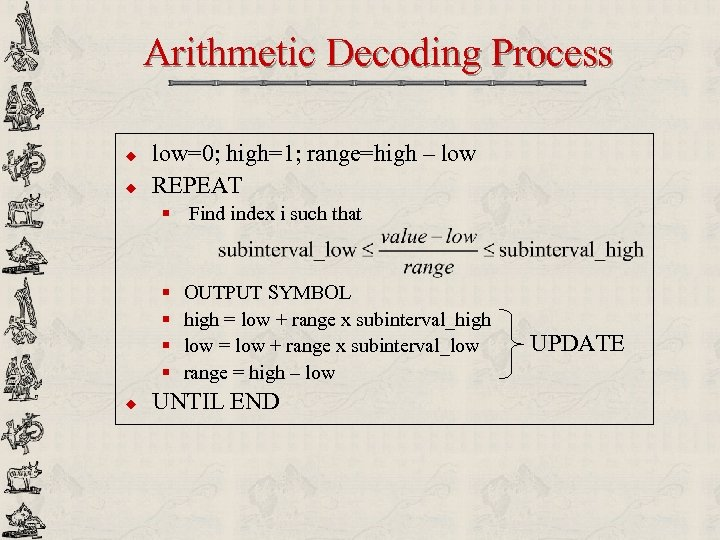Arithmetic Decoding Process u u low=0; high=1; range=high – low REPEAT § Find index i such that § § u OUTPUT SYMBOL high = low + range x subinterval_high low = low + range x subinterval_low range = high – low UNTIL END UPDATE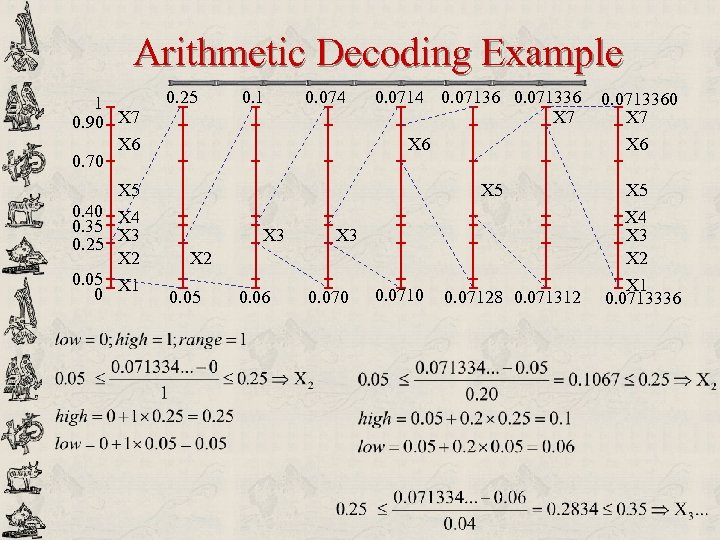Arithmetic Decoding Example 1 0. 90 X 7 X 6 0. 70 0. 25 0. 1 0. 074 0. 07136 0. 071336 X 7 X 6 X 5 0. 40 X 4 0. 35 X 3 0. 25 X 2 0. 05 X 1 0 X 6 X 5 X 3 0. 06 X 3 0. 070 X 5 X 4 X 3 X 2 0. 05 0. 0713360 X 7 0. 0710 0. 07128 0. 071312 X 1 0. 0713336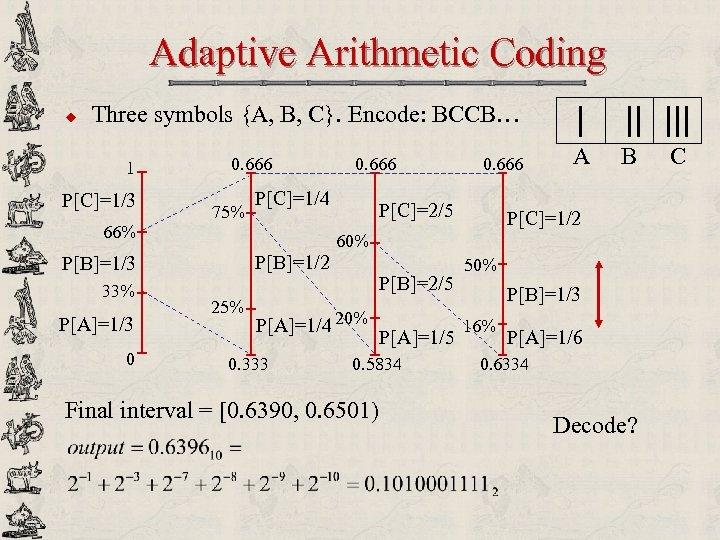Adaptive Arithmetic Coding u Three symbols {A, B, C}. Encode: BCCB… 1 P[C]=1/3 66% 0. 666 75% 33% P[A]=1/3 0 P[C]=1/4 P[B]=1/2 P[B]=1/3 25% 0. 666 P[C]=2/5 A B P[C]=1/2 60% P[B]=2/5 P[A]=1/4 20% 0. 333 0. 666 P[A]=1/5 0. 5834 Final interval = [0. 6390, 0. 6501) 50% P[B]=1/3 16% P[A]=1/6 0. 6334 Decode? C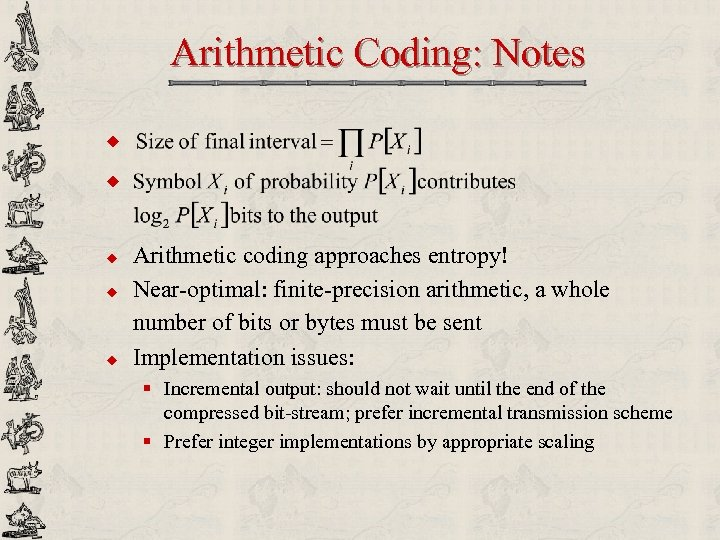Arithmetic Coding: Notes u u u Arithmetic coding approaches entropy! Near-optimal: finite-precision arithmetic, a whole number of bits or bytes must be sent Implementation issues: § Incremental output: should not wait until the end of the compressed bit-stream; prefer incremental transmission scheme § Prefer integer implementations by appropriate scaling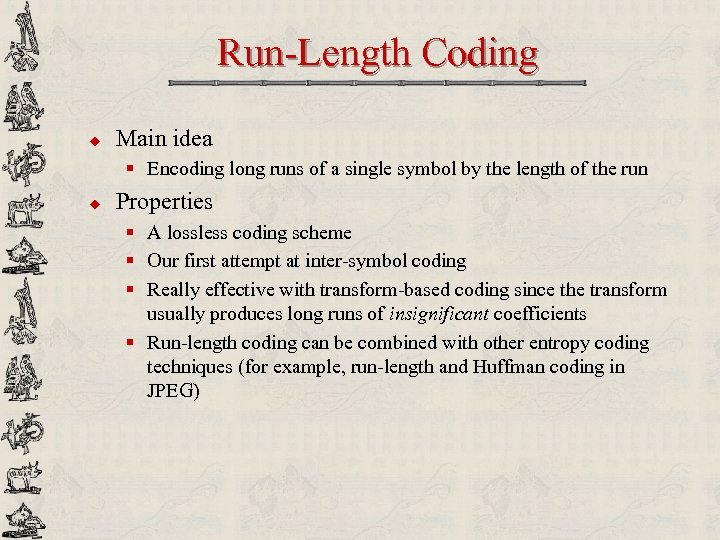Run-Length Coding u Main idea § Encoding long runs of a single symbol by the length of the run u Properties § A lossless coding scheme § Our first attempt at inter-symbol coding § Really effective with transform-based coding since the transform usually produces long runs of insignificant coefficients § Run-length coding can be combined with other entropy coding techniques (for example, run-length and Huffman coding in JPEG)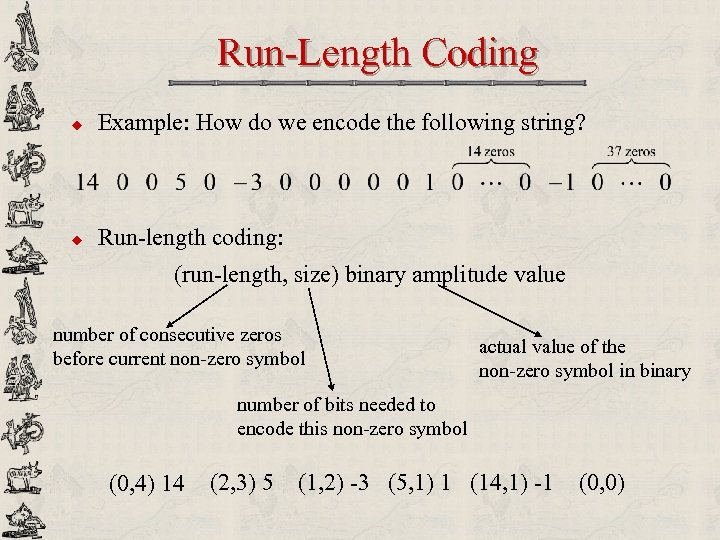Run-Length Coding u Example: How do we encode the following string? u Run-length coding: (run-length, size) binary amplitude value number of consecutive zeros before current non-zero symbol actual value of the non-zero symbol in binary number of bits needed to encode this non-zero symbol (0, 4) 14 (2, 3) 5 (1, 2) -3 (5, 1) 1 (14, 1) -1 (0, 0)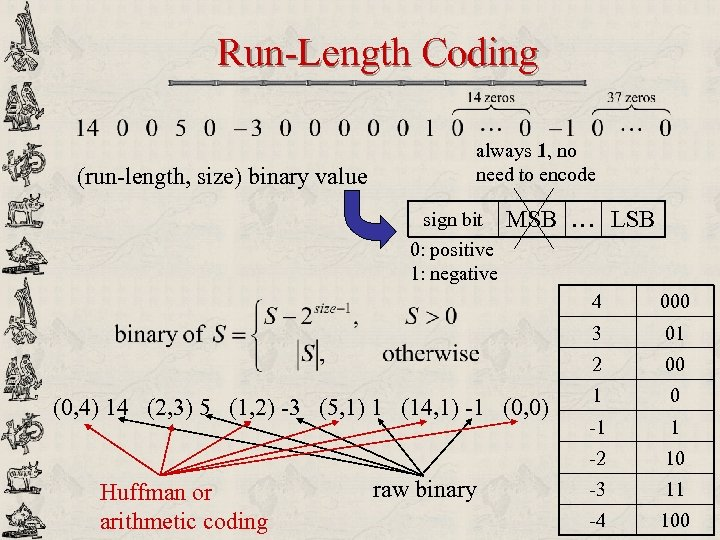Run-Length Coding (run-length, size) binary value always 1, no need to encode sign bit 0: positive 1: negative MSB … LSB 4 3 raw binary 00 1 0 -1 1 -2 Huffman or arithmetic coding 01 2 (0, 4) 14 (2, 3) 5 (1, 2) -3 (5, 1) 1 (14, 1) -1 (0, 0) 000 10 -3 11 -4 100